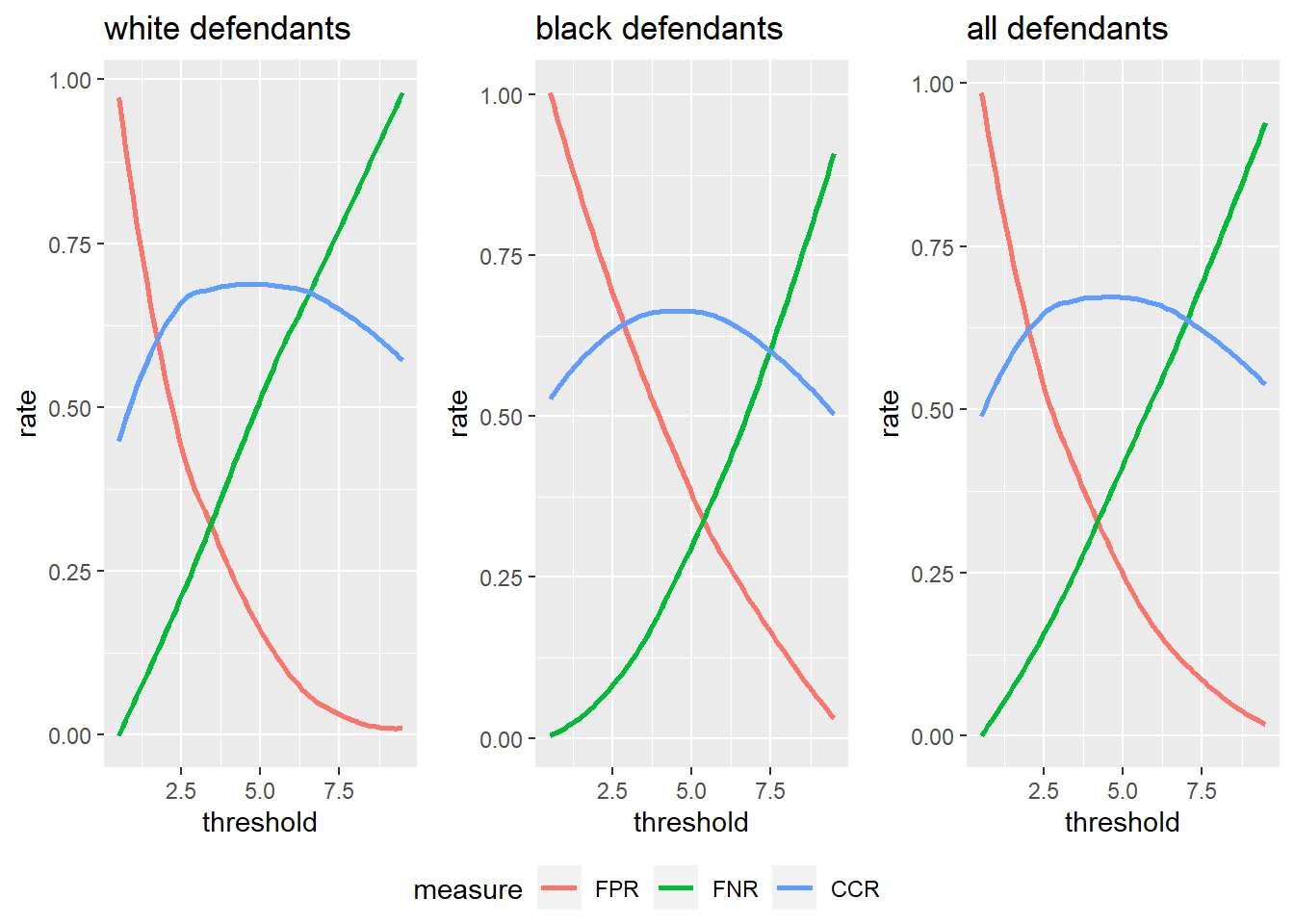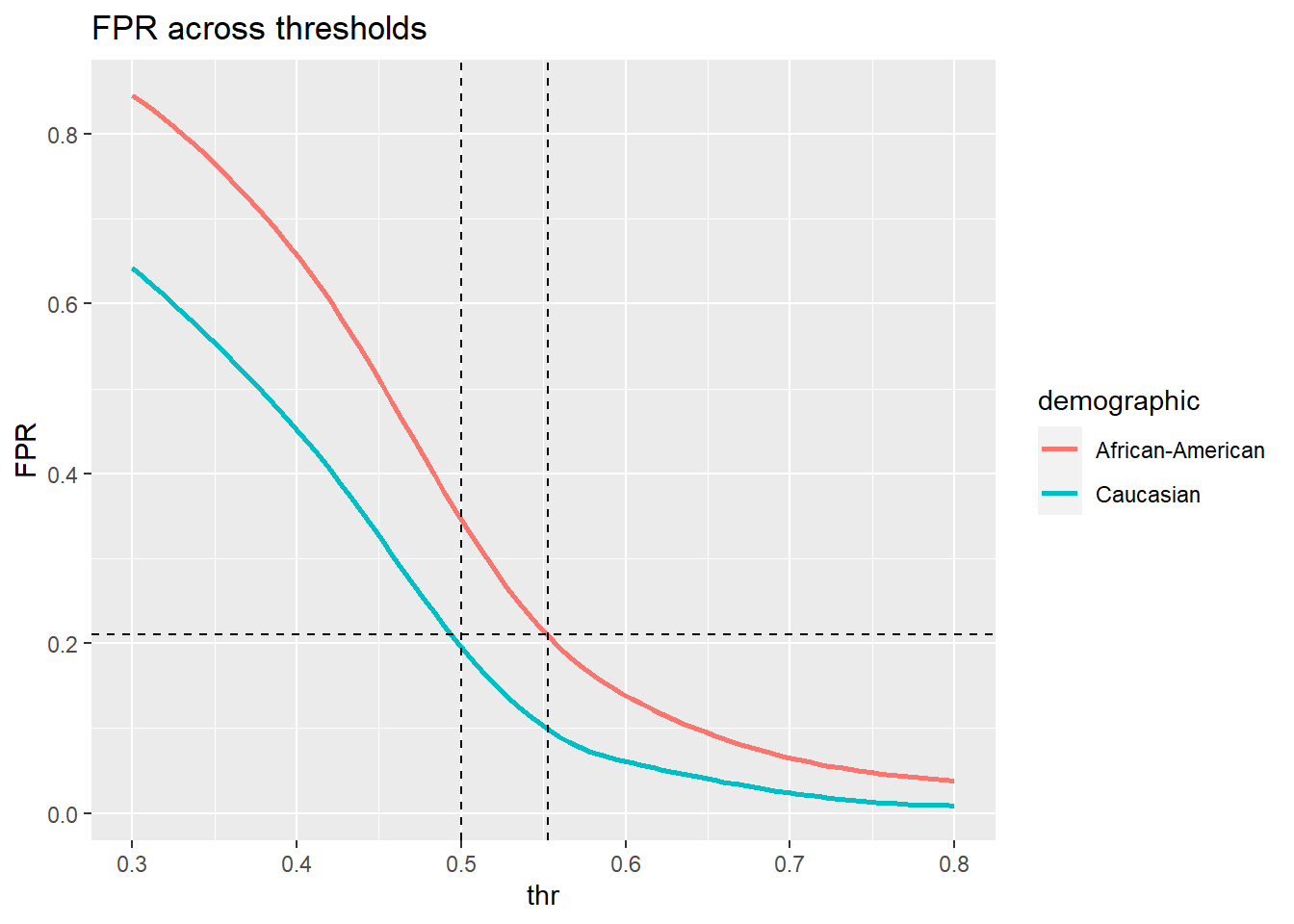## 21.3 Lab: Classifier performance & fairness

See previous lab for notes.

library(tidyverse)
library(reshape2)
library(tidyverse)
set.seed(0)
# write_csv(data, "compas-scores-two-years.csv")
data <- read_csv("compas-scores-two-years.csv")

See previous lab for notes.

# Add random vector
data <- data %>% mutate(random = sample(1:nrow(data)))
# Q: What happens here?

# First 5000 observations as training data
data_train <- data %>% filter(random >=1 & random<=5000)

# 1000 observations as test data
data_test <- data %>% filter(random >=5001 & random<=6000)

# 1000 observations as validation data
data.valid <- data %>% filter(random >=6001 & random<=7000)

See previous lab for notes.

fit <- glm(is_recid ~ age + priors_count,
family = binomial,
data = data_train)
#fit$coefficients summary(fit) ## ## Call: ## glm(formula = is_recid ~ age + priors_count, family = binomial, ## data = data_train) ## ## Deviance Residuals: ## Min 1Q Median 3Q Max ## -3.1159 -1.0670 -0.5752 1.1014 2.5503 ## ## Coefficients: ## Estimate Std. Error z value Pr(>|z|) ## (Intercept) 1.014762 0.097015 10.46 <2e-16 *** ## age -0.047415 0.002838 -16.71 <2e-16 *** ## priors_count 0.162316 0.008208 19.77 <2e-16 *** ## --- ## Signif. codes: 0 '***' 0.001 '**' 0.01 '*' 0.05 '.' 0.1 ' ' 1 ## ## (Dispersion parameter for binomial family taken to be 1) ## ## Null deviance: 6923.1 on 4999 degrees of freedom ## Residual deviance: 6200.0 on 4997 degrees of freedom ## AIC: 6206 ## ## Number of Fisher Scoring iterations: 4 ### 21.3.1 Initial evaluation of the COMPAS scores Here, we are computing the False Positive Rage (FPR), False Negative Rate (FNR) and the correct classification rate (CCR) for different populations.To do so we use the validation data data.valid. First, we’ll define functions to compute the quantities needed. thr in the functions below is the threshold. is_recid incates whether someone recidivated. # Function: False Positive Rate get.FPR <- function(data.set, thr){ return( # display results sum((data.set$decile_score >= thr) &
(data.set$is_recid == 0)) # Sum above threshold AND NOT recidivate / sum(data.set$is_recid == 0) # Sum NOT recividate
)
}
# Share of people over the threshold that did not recidivate
# of all that did not recidivate (who got falsely predicted to
# recidivate)

# Q: Explain the function below!

# Function: False Negative Rate
get.FNR <- function(data.set, thr){
return(sum((data.set$decile_score < thr) & (data.set$is_recid == 1)) # ?
/
sum(data.set$is_recid == 1)) # ? } # Q: Explain the function below! # Function: Correct Classification Rate get.CCR <- function(data.set, thr){ return(mean((data.set$decile_score >= thr) # ?
==
data.set$is_recid)) # ? } Compute the data sets we need: data.valid.b <- data.valid %>% filter(race == "African-American") data.valid.w <- data.valid %>% filter(race == "Caucasian") Now, we can compute the numbers: thr <- 5 fps <- c(get.FPR(data.valid.b, 5), get.FPR(data.valid.w, 5), get.FPR(data.valid, 5)) fns <- c(get.FNR(data.valid.b, 5), get.FNR(data.valid.w, 5), get.FNR(data.valid, 5)) ccr <- c(get.CCR(data.valid.b, 5), get.CCR(data.valid.w, 5), get.CCR(data.valid, 5)) rates <- data.frame(FPR = fps, FNR = fns, CCR = ccr) rownames(rates) = c("black", "white", "all") rates FPR FNR CCR black 0.4336283 0.2539062 0.6618257 white 0.2067308 0.4683544 0.6803279 all 0.2992278 0.3692946 0.6670000 We can see that the scores do not satisfy false positive parity and do not satisfy false negative parity. The scores do satisfy classification parity. Demographic parity is also not satisfied. ### 21.3.2 False positives negatives and correct classification Let’s now obtain the FPR, FNR, and CCR for our own predictive logistic regression model, using the threshold $$0.5$$. In the functions below we first generate predicted values for our outcome is_recid using the predict() function. Then we create a vector pred indicating whether those predicted values are above the threshold thr. Then we basically do the same as above, however, now we don’t use the COMPAS scores stored in decile_score but use our own predicted values. The predicted values are between 0 and 1 and not deciles as in decile_score. Hence, we have to change thr from 5 to 0.5. # Functions to compute FPR, FNR, CCR using predicted values for # recidivism variable get.FPR.fit <- function(thr, data.set, fit){ pred <- predict(fit, newdata = data.set, type = "response") > thr return(sum((pred == 1) & (data.set$is_recid == 0))/sum(data.set$is_recid == 0)) } get.FNR.fit <- function(thr, data.set, fit){ pred <- predict(fit, newdata = data.set, type = "response") > thr return(sum((pred == 0) & (data.set$is_recid == 1))/sum(data.set$is_recid == 1)) } get.CCR.fit <- function(thr, data.set, fit){ pred <- predict(fit, newdata = data.set, type = "response") > thr return(mean(pred == data.set$is_recid))
}

# Create data subsets
data_train.b <- data_train %>% filter(race == "African-American")
data_train.w <- data_train %>% filter(race == "Caucasian")
data.valid.b <- data.valid %>% filter(race == "African-American")
data.valid.w <- data.valid %>% filter(race == "Caucasian")
thr <- .5 # define threshold

# Get results for training data
demo.perf.dat.train <- data.frame(FP =  c(get.FPR.fit(thr, data_train, fit),
get.FPR.fit(thr, data_train.w, fit),
get.FPR.fit(thr, data_train.b, fit)),
FN =  c(get.FNR.fit(thr, data_train, fit),
get.FNR.fit(thr, data_train.w, fit),
get.FNR.fit(thr, data_train.b, fit)),
CCR =  c(get.CCR.fit(thr, data_train, fit),
get.CCR.fit(thr, data_train.w, fit),
get.CCR.fit(thr, data_train.b, fit)))

demo.perf.dat.train
FP FN CCR
0.2736357 0.3757298 0.6774000
0.2068618 0.5289017 0.6607249
0.3604149 0.2777390 0.6853282
# Get results for validation data
demo.perf.dat.valid <- data.frame(FP =  c(get.FPR.fit(thr, data.valid, fit),
get.FPR.fit(thr, data.valid.w, fit),
get.FPR.fit(thr, data.valid.b, fit)),
FN =  c(get.FNR.fit(thr, data.valid, fit),
get.FNR.fit(thr, data.valid.w, fit),
get.FNR.fit(thr, data.valid.b, fit)),
CCR =  c(get.CCR.fit(thr, data.valid, fit),
get.CCR.fit(thr, data.valid.w, fit),
get.CCR.fit(thr, data.valid.b, fit)))

demo.perf.dat.valid
FP FN CCR
0.2162162 0.3713693 0.7090000
0.1634615 0.4873418 0.6967213
0.3097345 0.2617188 0.7157676

It appears that there is basically no overfitting.

### 21.3.3 Altering the threshold

We can also explore how changing the threshold influences the false positive, false negative, and correct classification rates for the different groups (black, white, all). Below we write the function get.rates to get all the rates for a particular group for different threshold values. Then we write the plot.rates function to plot the rates for different groups.

# Get rates for different threshold values
# Function
get.rates <- function(thr, data.set){
return(c(get.FPR(data.set, thr), get.FNR(data.set, thr), get.CCR(data.set, thr)))
}

# Execute function
thrs <- seq(0.5, 9.5, 1)
rates.w <- sapply(thrs, FUN=get.rates, data.valid.w)
rates.b <- sapply(thrs, FUN=get.rates, data.valid.b)
rates.all <- sapply(thrs, FUN=get.rates, data.valid)

# Function: plot rates
plot.rates <- function(thrs, rates, caption){
rates.df <- data.frame(threshold = thrs, FPR = rates[1,], FNR = rates[2,], CCR = rates[3,])
rates.df.tidy <- melt(rates.df, 1) %>% select(threshold = threshold, measure = variable, rate = value)
ggplot(rates.df.tidy, mapping = aes(x = threshold, y = rate, color = measure)) +
geom_smooth(se = F, method = "loess") + labs(title = caption)
}

We can now get three figures for the different demographics (let’s forego using facets again):

library(ggpubr)
p1 <- plot.rates(thrs, rates.w, "white defendants")
p2 <- plot.rates(thrs, rates.b, "black defendants")
p3 <- plot.rates(thrs, rates.all, "all defendants")
plots_combined <- ggarrange(p1, p2, p3,
common.legend = TRUE,
legend = "bottom",
ncol = 3,
nrow = 1
)
plots_combinedWe basically want to know how to we would need to adapt the thresholds for black and whites so that their false positive rates are at parity. In other words we would like to find the thresholds for which the false positive rates are at parity. Let’s see what the rates are for different thresholds.

thr <- c(0.3, 0.4, 0.51, 0.52, 0.53, 0.54, 0.55, 0.553, 0.555, 0.558, 0.56, 0.57, 0.58, 0.59, 0.7, 0.8)
fp.rates <- data.frame(thr = thr,
FP.b  = sapply(thr, get.FPR.fit, data_train.b, fit),
FP.w = sapply(thr, get.FPR.fit, data_train.w, fit))

fp.rates
thr FP.b FP.w
0.300 0.8452895 0.6417760
0.400 0.6611927 0.4550959
0.510 0.3137424 0.1725530
0.520 0.2921348 0.1523713
0.530 0.2601556 0.1352170
0.540 0.2342264 0.1180626
0.550 0.2152118 0.1019173
0.553 0.2091616 0.0968718
0.555 0.2065687 0.0948537
0.558 0.1961971 0.0918264
0.560 0.1944685 0.0908174
0.570 0.1780467 0.0807265
0.580 0.1642178 0.0706357
0.590 0.1495246 0.0655903
0.700 0.0734659 0.0232089
0.800 0.0380294 0.0090817

We need to tweak the threshold for black defendants just a little:

plot.FPR <- function(fp.rates){
colnames(fp.rates) <- c("thr", "African-American", "Caucasian")
df.melted <- melt(fp.rates, "thr")
colnames(df.melted) <- c("thr", "demographic", "FPR")
ggplot(df.melted) +
geom_smooth(mapping=aes(x = thr, y = FPR, color = demographic), method = "loess", se = F) +
geom_vline(xintercept = 0.5, linetype = "dashed") +
geom_vline(xintercept = 0.553, linetype = "dashed") +
geom_hline(yintercept = 0.211, linetype = "dashed") +
ggtitle('FPR across thresholds')
}

plot.FPR(fp.rates)thr = 0.553 seems about right.

Now the white and black demographic would be at parity. We’ll compute the correct classification rate on the validation set.

n.correct <- sum(data_train.b$is_recid == (predict(fit, newdata = data_train.b, type = "response") > 0.553)) + sum(data_train.w$is_recid == (predict(fit, newdata = data_train.w, type = "response") > 0.5))
n.total <- nrow(data_train.b) + nrow(data_train.w)

n.correct/n.total
##  0.6541072

(Note that we ignored everyone who wasn’t white or black. That’s OK to do, but including other demographics (in any way you like) is OK too).